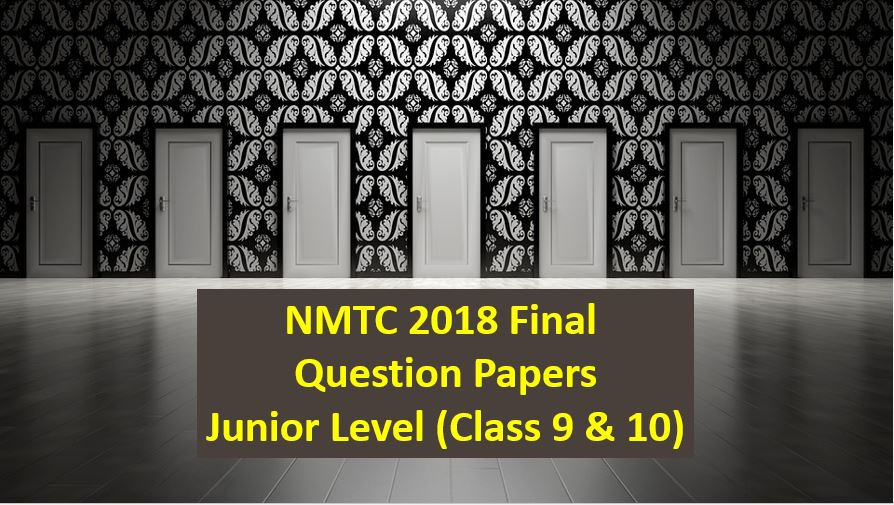Saturday, December 4, 2021
Home > Latest Announcement > NMTC 2018 Final Stage Class 9 and 10 Questions With Solutions

# NMTC 2018 Final Stage Class 9 and 10 Questions With Solutions# NMTC 2018 Final Stage Class 9 and 10 Question Paper With Solutions

In this post, you will get the following NMTC 2018 Final Stage Class 9 and 10 Questions with Solutions. Solutions will be uploaded soon.

## NMTC 2018 Final Stage Class 9 and 10 Questions With Solutions Question No 1:

ABC is a  right triangle with BC as a hypotenuse. The medians are drawn to BC and AC are perpendicular to each other. if AB has length 1 cm, find the area of the triangle ABC.

ANSWER:cm2.

Solution:

## NMTC 2018 Final Stage Class 9 and 10 Questions With Solutions Question No 2 (a):

Find the smallest positive integer such that it has exactly 100 different positive integer divisors including 1 and itself.

Solution:

## NMTC 2018 Final Stage Class 9 and 10 Questions With Solutions Question No 2 (b):

A rectangle can be divided into n equal squares. The same rectangle can also be divided into (n + 76) equal squares. Find the value of n.

Solution:

## NMTC 2018 Final Stage Class 9 and 10 Question Paper With Solutions Question No 3:

Prove that 1n + 2n + 3n + … + 15n is divisible by 480 for all odd n >= 5.

Solution:

## NMTC 2018 Final Stage Class 9 and 10 Question Paper With Solutions Question No 4:

Is it possible to have 19 lines in a plane such that no three lines have a common point and they have exactly 95 points of intersections.

Solution:

## NMTC 2018 Final Stage Class 9 and 10 Question Paper With Solutions Question No 5:

In a trapezium ABCD with AB parallel to CD, the diagonals intersect at P. The area of triangle ABP is 72 cm2 and the area of triangle CDP is 50 cm2. Find the area of trapezium.

Solution:

## NMTC 2018 Final Stage Class 9 and 10 Question Paper With Solutions Question No 6:

Let a < b < c be three positive integers. Prove that among any 2c consecutive positive integers there exists three different numbers x, y, z such that abc divides xyz.

Solution:

## NMTC 2018 Final Stage Class 9 and 10 Question Paper With Solutions Question No 7 (a):

Let m and n be two positive integers. If m3 + n3 is the square of an integer, then prove that (m + n) is not a product of two different prime numbers.

Solution:

## NMTC 2018 Final Stage Class 9 and 10 Question Paper With Solutions Question No 7 (b):

a, b, c are three real numbers such that ab + bc + ca = -1. Prove that a2 + 5b2 + 8c2 >= 4.

Solution:

## NMTC 2018 Final Stage Class 9 and 10 Question Paper With Solutions Question No 8:

ABCD is a quadrilateral in a circle whose diagonals intersect at right angles. Through O the center of the circle GOG’ and HOH’ are drawn parallel to AC and BD respectively, meeting AB and CD in G, H and DC, AB produced in G’ and H’. Prove that GH. G’H’ are parallel to BC and AD respectively.

Solution: